# Time complexity of Algorithms

Sometimes, there is more than one way to solve a particular problem. So It is important to find the most efficient way to solve the problem. Analysis of algorithms is very important while solving problems in computer science. To solve a problem in an efficient way we need to learn how to compare the performance of different algorithms for a particular problem and choose the most efficient one to solve it. Time complexity depends on various software and hardware parts of the computer but while analyzing an algorithm, we generally consider time complexity and space complexity. The time complexity of an algorithm is the time taken by an algorithm to run as a function of the length of the input. And, the Space complexity of an algorithm is the amount of space or memory required by an algorithm to run as a function of the length of the input.

# What is Time complexity?

The time complexity is the number of operations an algorithm performs to complete its task with respect to the input size or the time taken by an algorithm to run as a function of the length of the input. The algorithm that performs the task in the smallest number of operations or takes less time is considered the most efficient one.

Suppose we are having one problem and we wrote two algorithms for the same problem. Now, we need to choose one of these two algorithms. How will you do that?

For example, we have to search for a particular number within a given array then we can search the number in two ways either by linear search or by binary search.

First, let’s see the example of a linear search through code.

Here in the given code, the linear search algorithm will compare each element of the array with sear_digit and the result will be true when the digit will be found in the array. There will be 10 comparisons in case the search_digit is not present. So the maximum number of operations is equal to the length of the given array. Hence the time complexity of the Linear search algorithm is O(n).

let’s see the example of a Binary search through code.

Here in the given code, the Binary search algorithm is Searching a sorted array by repeatedly dividing the search interval in half. Begin with an interval covering the whole array. If the value of the search_digit is less than the item in the middle of the interval, decreases the interval to the lower half. Otherwise decreases it to the upper half. Repeatedly check until the value is found or the interval is empty. So it will take 4 comparisons to find 1 or 10 which is approx log10(to the base 2). So the time complexity of the algorithm is log(n). So we can say that a binary search is the best algorithm for searching.

# Representation Of time complexity

There are three asymptotic notations that are used to represent the time complexity of an algorithm. They are:

1.Θ Notation

2.Big O Notation

3.Ω Notation

# Θ Notation

The Θ Notation is used to defines an upper bound and a lower bound, and our algorithm will be lie in between these bounds i.e it finds the average bound of an algorithm. So, if a function is g(n), then the theta representation is shown as:

`Θ(g(n)) = { f(n): there exist positive constants c1, c2 and n0          such that 0 ≤ c1g(n) ≤ f(n) ≤ c2g(n) for all n ≥ n0 }`

So in the above expression theta of g(n) is defined as a set of all the functions f(n) for which there exists some positive constants c1, c2, and n0 such that c1*g(n) is less than or equal to f(n) and f(n) is less than or equal to c2*g(n) for all n that is greater than or equal to n0.

For example:

`if f(n) = 2n² + 3n + 1and g(n) = n²then for c1 = 2, c2 = 6, and n0 = 1, we can say that f(n) = Θ(n²)`

# Big O Notation

The Big O notation used to defines the upper bound of an algorithm i.e. This is the maximum possible time of the algorithm.

Big O notation is the most used notation for the time complexity of an algorithm. For example, if a function is g(n), then the big O representation of g(n) is shown as:

`O(g(n)) = { f(n): there exist positive constants c and n0          such that 0 ≤ f(n) ≤ cg(n) for all n ≥ n0 }`

In the above example, Big O of g(n) is defined as a set of functions f(n) for which there exist some constants c and n0 such that f(n) is greater than or equal to 0 and f(n) is smaller than or equal to c*g(n) for all n greater than or equal to n0.

`if f(n) = 2n² + 3n + 1and g(n) = n²then for c = 6 and n0 = 1, we can say that f(n) = O(n²)`

# 3.Ω Notation

The Ω notation denotes the minimum time taken by the algorithm, the time taken by the algorithm can’t be lower than this.

The Ω notation denotes lower bound of an algorithm.For example, if a function is g(n), then the omega representation is shown as:

`Ω(g(n)) = { f(n): there exist positive constants c and n0           such that 0 ≤ cg(n) ≤ f(n) for all n ≥ n0 }`

The above expression can be read as omega of g(n) is defined as a set of all the functions f(n) for which there exist some constants c and n0 such that c*g(n) is less than or equal to f(n), for all n greater than or equal to n0.

`if f(n) = 2n² + 3n + 1and g(n) = n²then for c = 2 and n0 = 1, we can say that f(n) = Ω(n²)`

# Conclusion

In this blog, we learned about the time complexity of an algorithm. We saw how the time complexity is used to analyse the efficiency of an algorithm. We also saw the different ways of representation of the time complexity.That’s it for this article. Hope you learned something new today.

--

--

--

## More from Ajit Kumar Singh

Love podcasts or audiobooks? Learn on the go with our new app.

## Ridge regression, LASSO, Elastic Net## Is Fear of Math Holding You Back?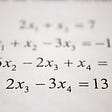## Covariance and Correlation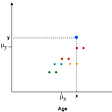## Check if a Binary Tree is a Subtree of another Binary Tree## So…an ellipse is a sine wave in disguise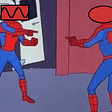## Probability theory: Bayes’ theorem## Primes in a Primary Text?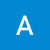## NSOperations and its practical usage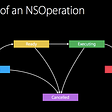## Getting, VirtualBox “drag and drop” feature and using “alt + Tab” keys to swith between Host…## Binary Incompatibility explained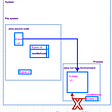## CS373 Spring 2022: Anthony Modica